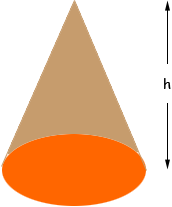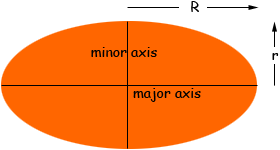Name: Richard Who is asking: Other Level of the question: All Question: I am trying to find the volume of a cone that is not round but oval. I am sure there is a way to measure this, but I have not found anything yet that I am willing to live with. Richard, There is a nice result in geometry which says that if you take a region in the plane which has an area, a point P not in the plane and form a "tent" by joining every point on the boundary of the region to the P then the volume of the tent is   1/3(the area of the region at the base)(the height of the tent) Your oval-based cone fits this description,and hence if you know the height you only need find the area of the oval. If the oval is an ellipse then it has a major axis and a minor axis. Let R be half the length of the major axis and let r be half the length of the minor axis,then the area of the ellipse isrR Hence if the base of your cone is an ellipse then the volume is   1/3rRh Penny